Latest Banking jobs   »

# Quantitative Aptitude Quiz For SIDBI Grade A Prelims 2023- 24th January

Direction (1-5) Please understand the data carefully and answer the following questions.
The table below shows the investment of five different persons, the time for which they invested and rate of interest per annum.
Note:
You have to only calculate the simple interest unless asked for compound interest.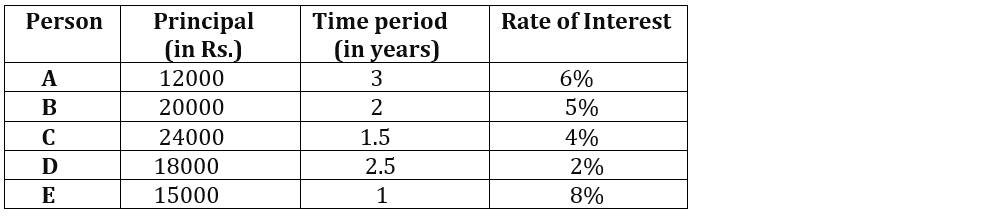Q1. Find the simple interest received by D is what percent less/more than the simple interest received by E?

(a)75%

(b)20%

(c)10%

(d)25%

(e)50%

Q2. Find the difference between the simple interest and compound interest received by B for the same amount at the same rate and time period?

(a) Rs.25

(b) Rs.75

(c)Rs.150

(d)Rs.100

(e)Rs.50

Q3. Find the respective ratio of simple interest received by C to the total amount received by A?

(a)7: 51

(b)5: 51

(c)6: 59

(d)8: 59

(e)3:52

Q4. Find the amount received by B is what more/less than the amount received by D? (in Rs.)

(a)2100

(b)3100

(c)2700

(d)3400

(e)1900

Q5. If interest received by F is 20% of the combined interest of D & E, Moreover, F invested the money for 2 years at 1% more than the rate at which C invested. Find the sum invested by F.

(a)Rs.8400

(b)Rs.9600

(c)Rs.9000

(d)Rs.8000

(e)Rs.9400

Direction (6-10): Please understand the data carefully and answer the following questions.

The following bar graph shows the number of days taken by five different persons individually, P, Q, R, S & T, to finish the work completely.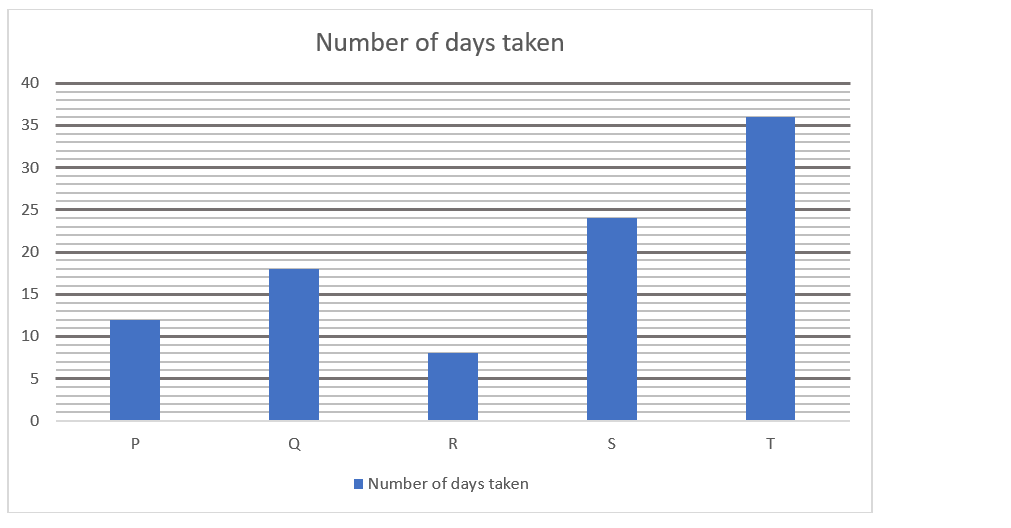Q6. If S and T started doing work together and T left after 6 days, then find the time taken by S to finish the remaining work? (in days)
(a)8
(b)10
(c)16
(d)12
(e)14
Q7. Find the difference between the number of days taken by R & S together and Q & T together to finish the whole work? (in days)
(a)1
(b)12
(c)6
(d)9
(e)3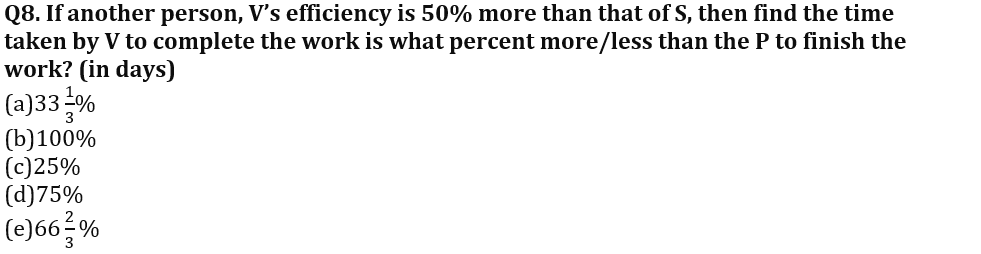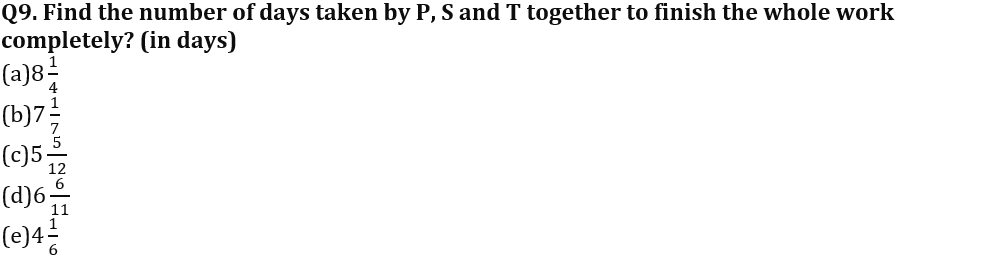Q10. If R and S decide to work together but alternately starting from S, then find the number of days taken to finish the work? (in days)
(a)8
(b)12
(c)16
(d)6
(e)10
Direction (11-15): Please understand the data carefully and answer the following questions.
The following table shows the length (in meters) and speed (in m/s) of five different trains, A, B, C, D & E.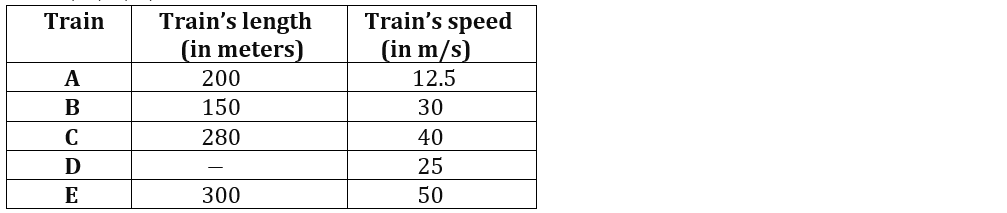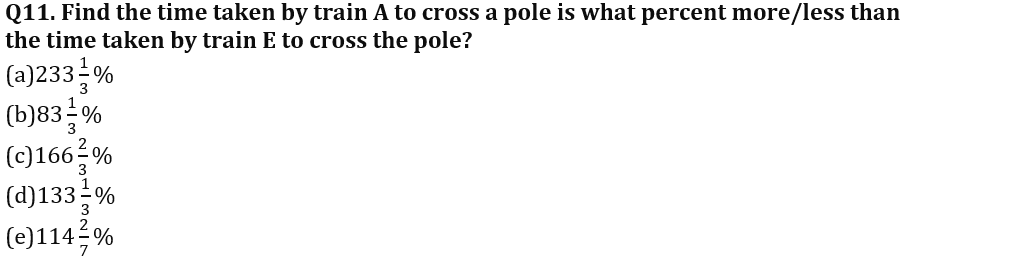Q12. If length of train D is 50% more than the length of train B, then find the respective ratio of time taken by train C to cross a pole to the time taken by train D to cross the pole.
(a)9:8
(b)8:7
(c)7:6
(d)9:5
(e)7:9

Q13. Find the time taken by train A and train E to completely cross each other, if they are moving in opposite direction? (in sec)
(a)10
(b)8
(c)12
(d)6
(e)15
Q14. If train F crosses a pole and platform in 8 seconds and 20 seconds respectively, then find the length of train F, given that length of platform is 240 meters?
(a)160 meter
(b)200 meter
(c)240 meter
(d)120 meter
(e)300 meter
Q15. Find the time taken by train B and train C to completely cross each other, if they are running in same direction? (in sec)
(a)39
(b)55
(c)47
(d)43
(e)51

Solutions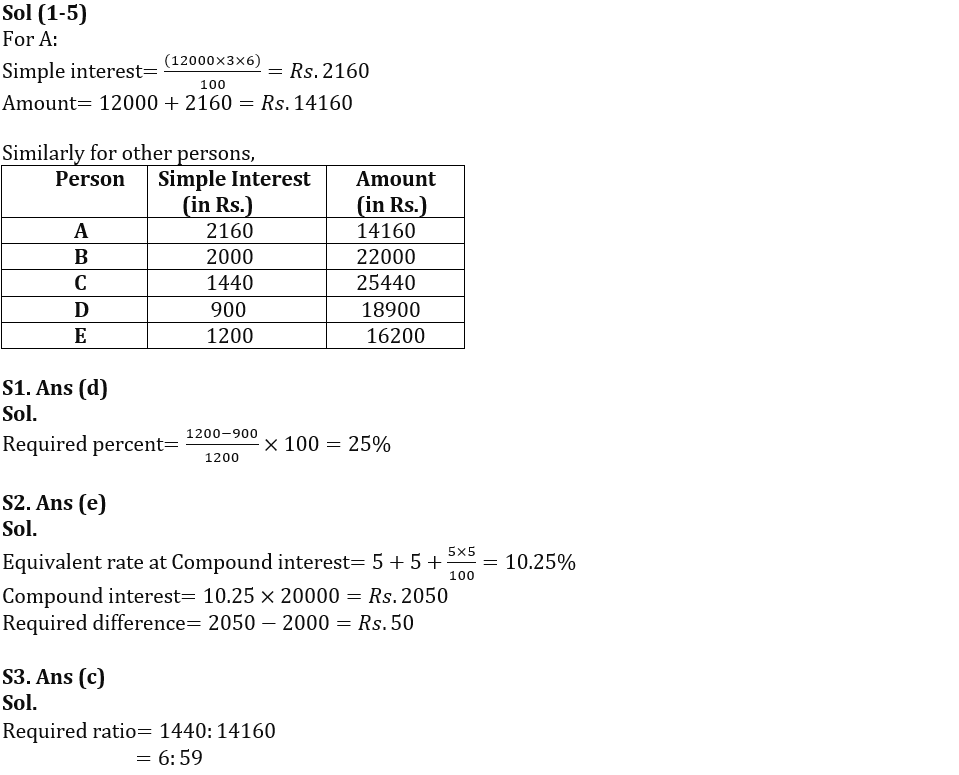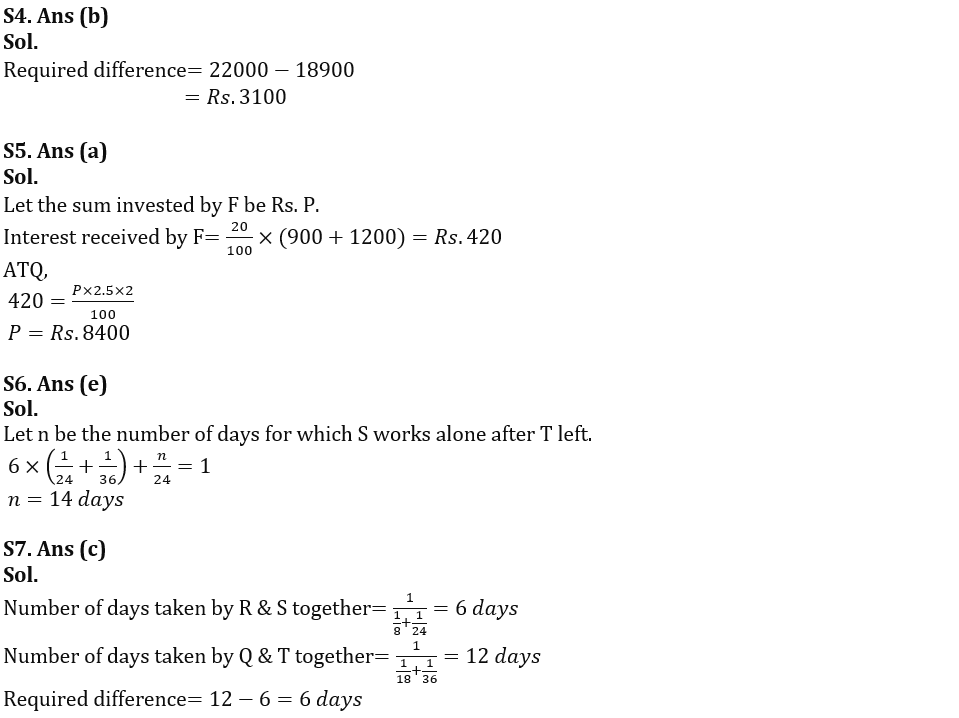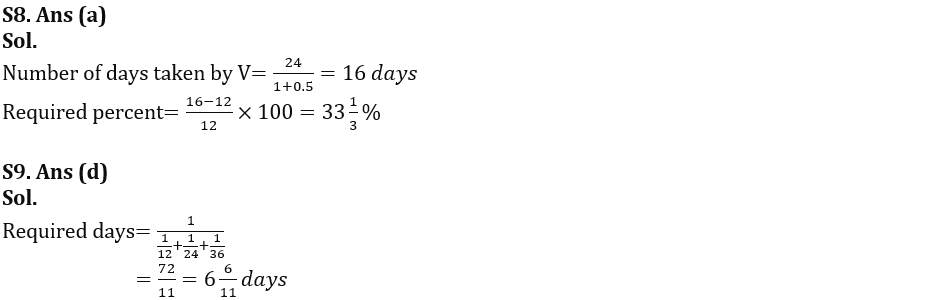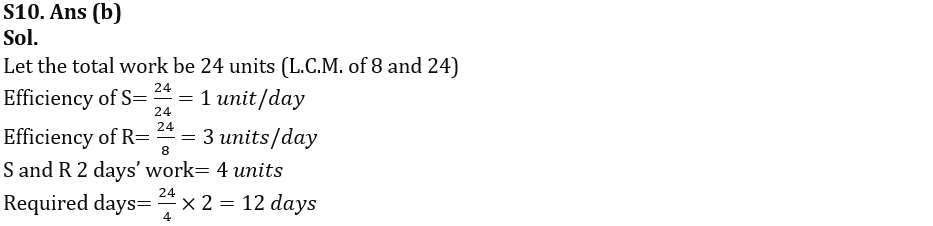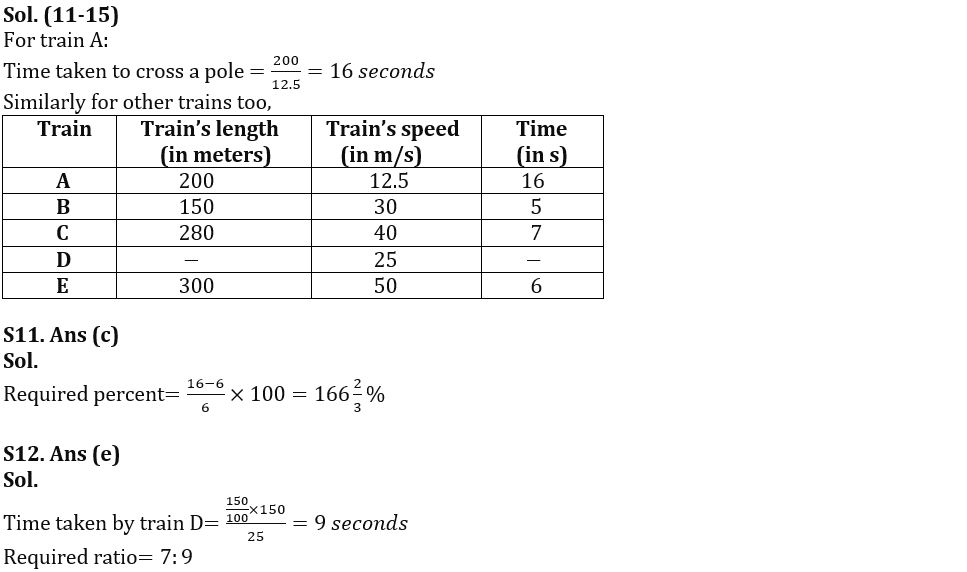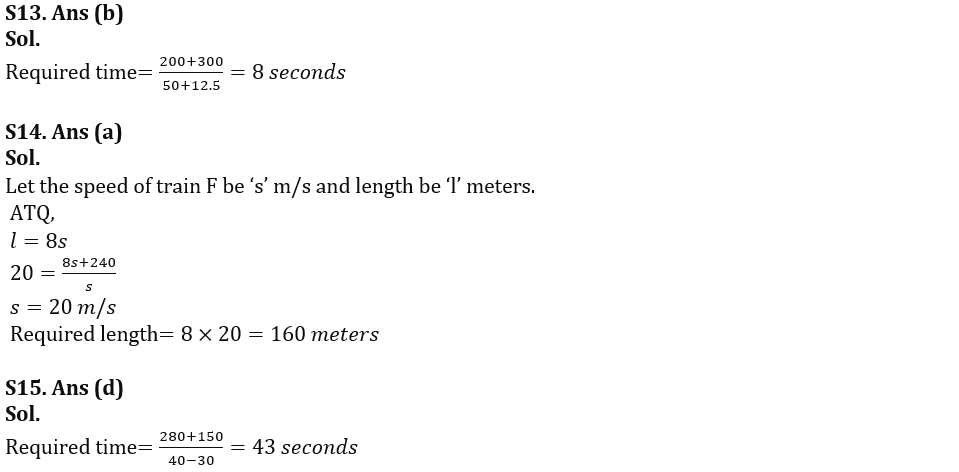## FAQs

### Quantitative Aptitude Quiz For SIDBI Grade A Prelims 2023- 21st January

The SIDBI Grade A Exam 2023 will be held on 28th January 2023.

#### Congratulations!